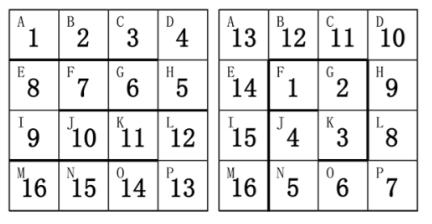# 蓝桥杯打卡第15天(2.16)

### 1.题目描述

``````/*每周的能量为该周符数的中位数
降雨量为7周能量的中位数，即第4周的中位数
求最大降雨量，只需使第4周的中位数尽可能的大
即使最后3周的每周的后4天和第4周的后3天为极大值，可得解
*/
#include <iostream>
using namespace std;
int main()
{
cout<<49-15<<endl;
return 0;``````

### 代码:

``````#include<stdio.h>
int main() {
int a = {0, 1, 2, 3, 4, 5, 6, 7, 8, 9, 10}, m, n, k;
scanf("%d", &m);
for (int i = 0; i < m; i++) {
scanf("%d", &n);

for (int j = 1; j < 11; j++) {
if (a[j] == n) {
for (k = j; k > 1; k--) {
a[k] = a[k - 1];
}
break;
}

}
a = n;
for (int j = 1; j < 11; j++) {
printf("%-4d", a[j]);
}
printf("\n");
}
return 0;

}``````

### 3.题目描述``````#include<stdio.h>
int a;
int sum;
int arr(int x, int y, int count) {
if (a[x][y] == 1)
return 1;
if (x < 1 || x > 4 || y < 1 || y > 4)
return 1;
if (count == 16) {
sum++;
return 1;
}
a[x][y] = 1;
arr(x - 1, y, count + 1);
arr(x + 1, y, count + 1);
arr(x, y - 1, count + 1);
arr(x, y + 1, count + 1);
a[x][y]=0;
return 1;
}
int main() {
arr(1, 1, 1);
arr(1, 2, 1);
arr(1, 3, 1);
arr(2, 2, 1);
printf("%d", sum * 4);
return 0;
}
``````

...全文
7 回复 打赏 收藏 转发到动态 举报蓝桥杯打卡15
【代码】蓝桥杯打卡15蓝桥杯15（Python版）(数论)
【代码】蓝桥杯15（Python版）(数论)蓝桥杯打卡（220118）蓝桥杯打卡（220119）蓝桥杯Python组打卡——Day14

30,229发帖与我相关我的任务• 近7日
• 近30日
• 至今